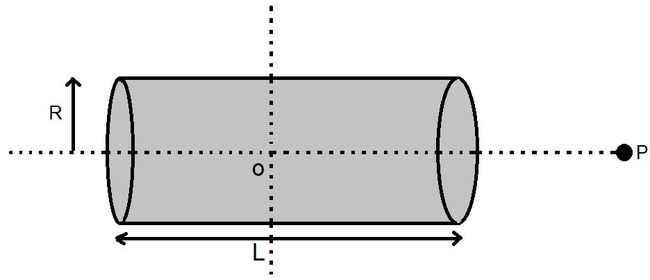# Electric Field at axis of Cylinder!A non-conducting solid cylindrical rod of length $L$ and radius $R$ has uniformly distributed charge $Q$. Find the electric field at point $P$, a distance $L$ from the center of the rod.

If $\large E = \frac{Q}{a \pi R^b L \epsilon_0}[ L+(\frac{L^c}{d} +R^e)^{f} - (\frac{gL^h}{i} +R^j)^{k} ]$

then, Find the sum of $(a+b+c+d+e+f+g+h+i+j+k)$.

Note : Fractions (ex $\dfrac 7 4$, $\dfrac 3 2$) must not be simplified and written as $\frac{1.75}{1} , \frac{1.5}{1}$

×